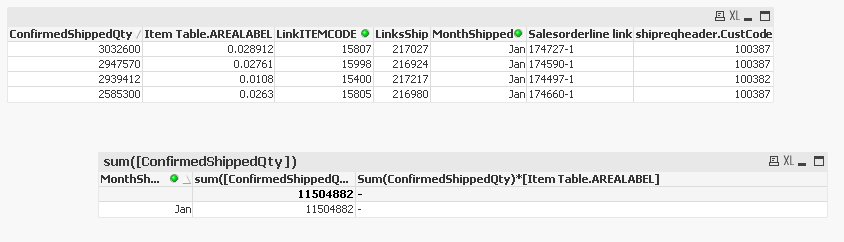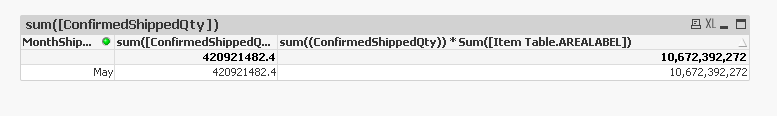# New to QlikView

Discussion board where members can get started with QlikView.

Highlighted
Contributor

## Straight table expression to multiply.

Everyone this is a strange one that should be simple however i cant get the desired result. See below i have used the expression as the labels on the straight table and table box to try and explain.

Okay basically the sum of the confirmedshippedqty is working but when i try to multiply by the itemtableAREALABEL the expression is null. It works when single items are selected.Working when single item selected.Hope this makes sense. Thanks Brett

1 Solution

Accepted Solutions
Contributor

## Re: Straight table expression to multiply.

Got it.

sum(aggr(sum((ConfirmedShippedQty)) * Sum([Item Table.AREALABEL]), [LinkITEMCODE]))

Thank you.

6 RepliesMVP

## Re: Straight table expression to multiply.

Hi,

Try this expression.

Sum(ConfirmedShippedQty * [Item Table.AREALABEL])

Or

Sum(ConfirmedShippedQty) * Sum([Item Table.AREALABEL])

Regards,

Kaushik Solanki

Valued Contributor

## Re: Straight table expression to multiply.

try

* sum(temtableAREALABE)

could be because they are at different granular levels..

Contributor

## Re: Straight table expression to multiply.

The expression is perfect but once i remove a dimension the sum changes see below.

Correct 9,664,320calculation is sum((ConfirmedShippedQty)) * Sum([Item Table.AREALABEL])

dont want to use a pivot table, require the second table but correct sum.MVP

## Re: Straight table expression to multiply.

Hi,

Try this

Sum(Aggr(sum((ConfirmedShippedQty)) * Sum([Item Table.AREALABEL]),LinkITEMCODE,MonthSip....))

Regards,

Kaushik Solanki

Contributor

## Re: Straight table expression to multiply.

Got it.

sum(aggr(sum((ConfirmedShippedQty)) * Sum([Item Table.AREALABEL]), [LinkITEMCODE]))

Thank you.

Contributor

Thank you